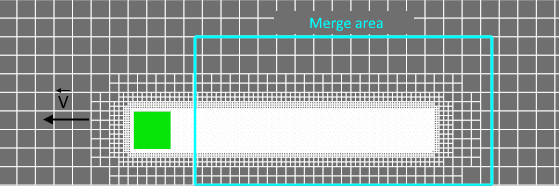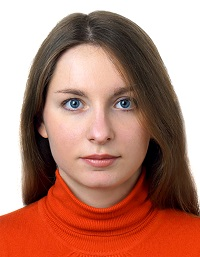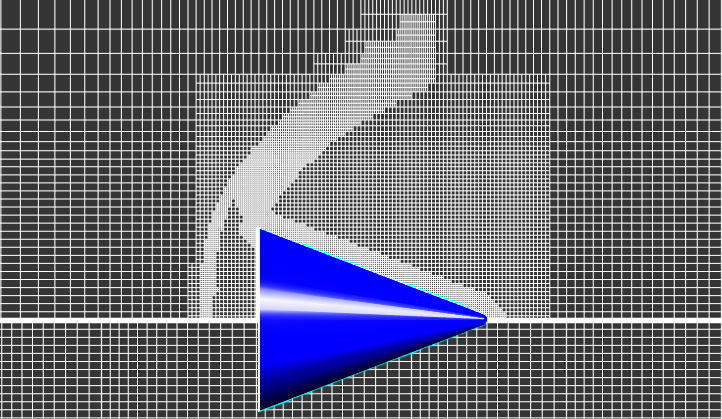### Article Index

The use of adaptation goes hand in hand with the study of grid convergence: determining for which combination of different adaptations the solution does not depend on further refinement of the computational grid.
Below we will discuss three techniques for using adaptations:

#### Setting a constant width of adaptation zone

The adaptation zone width is determined by a set of adaptation layers - a set of cells at the same refinement level.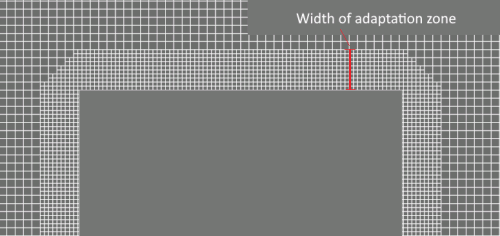It's good to remember that the width of the adaptation zone can change when you are specifying a sequential surface adaptation (Fig. Case 1). It is necessary for the width of the adaptable layer to be unchanged during the calculation if you want to resolve by grid only a studied area with maximum flow disturbances (Fig. Case 2). This requires changing the number of layers for each new level in the adaptation properties.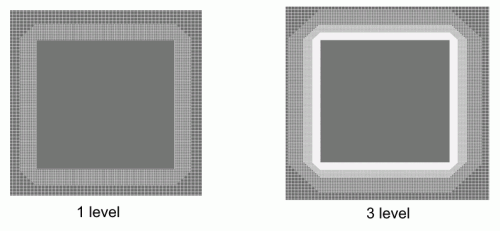Case 1. If the number of layers remains unchanged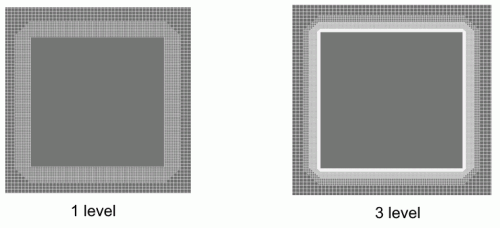Case 2. The number of layers is changed to maintain the thickness of the adaptation layer

Since the next level of adaptation splits the cell of the current level into two in each direction, it is convenient to use the following formula to calculate the number of layers for each level of adaptation, depending on its level. Let there be x layers at the initial adaptation level N-2. For the adaptation of level N, we'll define n1, n2, n3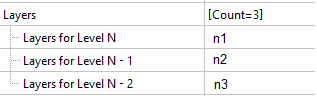from the equation: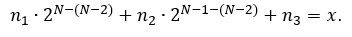#### Grid Convergence Automation

To automate the process of grid convergence investigation, it is convenient to apply adaptation using the formula or table that depends on time or step number.

With adaptation (that is, with a decrease in the size of computational grid) the time step decreases. This means that the time intervals between steps will become uneven.

##### how to set adaptation by formula editor

An example of setting adaptation of 3-5 levels, depending on the current time, on the surface of the Boundary condition "Wall":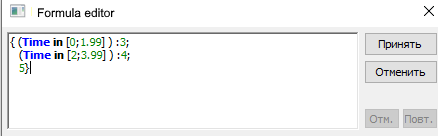##### how to set adaptation by a table

An example of setting adaptation of 3-5 levels, depending on the current time, on the surface of the Boundary condition "Wall":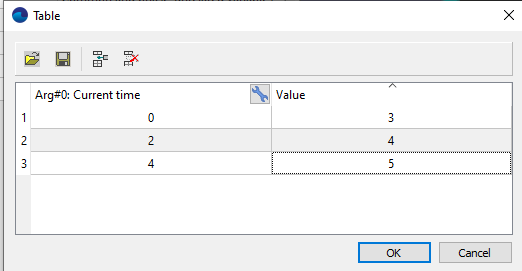##### RESULTs OF AUTOMATION OF THE PROCESS OF DETERMINING THE grid CONVERGENCE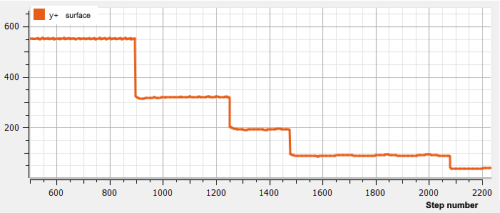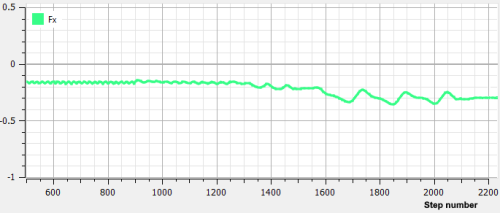Investigation of grid convergence: at Y+ = 44, the value of the longitudinal force is constant

(by time or by step)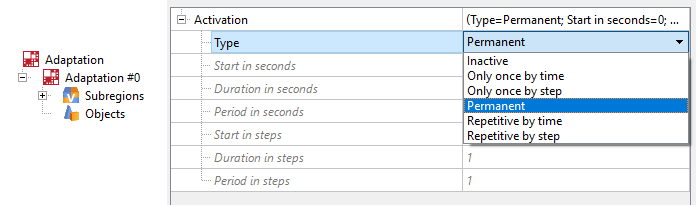The main advantage of using periodic adaptation is a significant decrease in calculation time. Periodic activation of adaptation does not impair the accuracy of calculation, but simply allows you to perform the adaptation process of the calculation cells less often: even setting the periodicity at only 20 steps will significantly speed up your calculation.

The use of a periodic type of activity is effective:

• for periodic application of adaptation to the solution in order to save computational time.
• when adapting the surface of moving bodies. Set merging for adaptation in the area behind the body to be performed periodically, and not at every iteration.
•